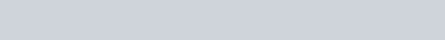# Linux chmod Command Examples

Filed Under: UNIX/Linux

In this guide, you will learn about the chmod command. Linux chmod command is one of the most commonly used commands especially by system administrators when assigning modifying file and folder permissions.

It’s usually used when installing and configuring various services and features in a Linux system. The command is usually used together with a set of octal notations or alphabetical characters to change file permissions.

## Linux File Permissions

Each file on the Linux systems bears a set of permissions. There are 3 permission types that are associated with a file.

1. Read permissions identified by ‘r
2. Write permissions identified by ‘w
3. Execute permissions identified by ‘x

To check the file permissions of any file, use the ls command and the `-l` option as shown below.

``\$ ls -l ``

For instance, to list permissions of file1.txt run the command.

``\$ ls -l file1.txt``

Sample OutputLet’s break down this output

The first hyphen (-) denotes that the permissions belong to a file, and not a folder. Permissions of a folder begins with a symbol d to denote a directory as shown.

``ls -l /var/www``

Sample OutputIn the output, the string `rwxr-xr-x` denotes the permissions of this file. This is divided into 3 sections as follows.

1. user permissions `(rwx)` – This represents the permissions of the owner or user who created the file. The `rwx` implies that the owner can read, write and execute the file.
2. Group permissions `(r-x)` -This represents permissions belonging to users in the file’s group. The r-x permissions imply that the group users can read and execute the file, but cannot write onto the file.
3. Other user permissions `(r-x)` – These are permissions for other users who neither belong to the two categories as discussed above. In this case, other users can only read the file.

## Permission Modes

There are 2 permission modes that can be passed to `chmod` command:

• Octal notation
• Alphabetical characters

### 1. Octal notation

Consider the permissions `rwx`. This implies the following:

``````
r=4
w=2
x=1
``````

so for instance, `rwx` is the equivalent of 4+2+1 which is equal to 7.

The corresponding numerical values to each of the alphabets are added to get the file permissions.

For example, a file with permissions `rwxr-xr--` will have an octal notation of `754`.

Here’s why:

Calculation

``````
rwx = 4+2+1 = 7
r-x = 4+0+1 = 5
r-- = 4+0+0 = 4
``````

As you may have noted, the hyphen takes the null value and is assigned 0.

Let’s take another example:

Say we have another file with permissions `rwx-rw-rw-`.

The octal notation would be calculated as follows:

Calculation

``````
rwx = 4+2+1 = 7
r-x = 4+2+0 = 6
r-- = 4+2+0 = 6
``````

Ultimately, this would give us `766` as the corresponding octal notation to `rwx-rw-rw-`.

### Changing file permissions with chmod command using octal notation

To change file permissions of a file use the syntax below.

``chmod [octal value] file-name``

For example, to change file permissions of a file file1.txt, to say `rw-r--r--` execute:

``chmod 644 file1.txt``

This is illustrated in the calculation below

``````
(user) rw- = 4+2+0 = 6
(group) r-- = 4+0+0 = 4
(others)r-- = 4+0+0 = 4
``````

### 2. Alphabetical Notation

In alphabetical notation, the write permissions are segmented into 3 sections with each section bearing the `rwx` sections.

From the left, we have the following notations:

``````
u  (user)
g  (group)
o  (others)
a (all)
``````

This is better illustrated below:### Changing file permissions with chmod command using alphabetical notation

To change file permissions using alphabetical notation, use the syntax below.

``chmod [user type(u/g/o/a)] [add/revoke(+/-)] [permission type(r/w/x)] ``

For instance to change permissions of the owner of a file to read and write, execute:

``chmod u+rw file1.txt``

To give write permissions to everyone, execute:

``chmod a+w file1.txt``

To remove the write permission for all other users, we run:

``chmod o-w file1.txt``

To change the permissions of a directory, we run:

``chmod [permission] [directory name]``

To change the permissions of a directory with its files and sub-directories recursively, we run:

``chmod -R [permission] [directory name]``

For example, to set the permission to `755` recursively to `/var/www/` diirectory execute the command.

``chmod -R 755 /var/www ``

We hope this article was insightful and helped you with the basics of the chmod command usage. As always, your feedback is most welcome.

1.Oleksey says: